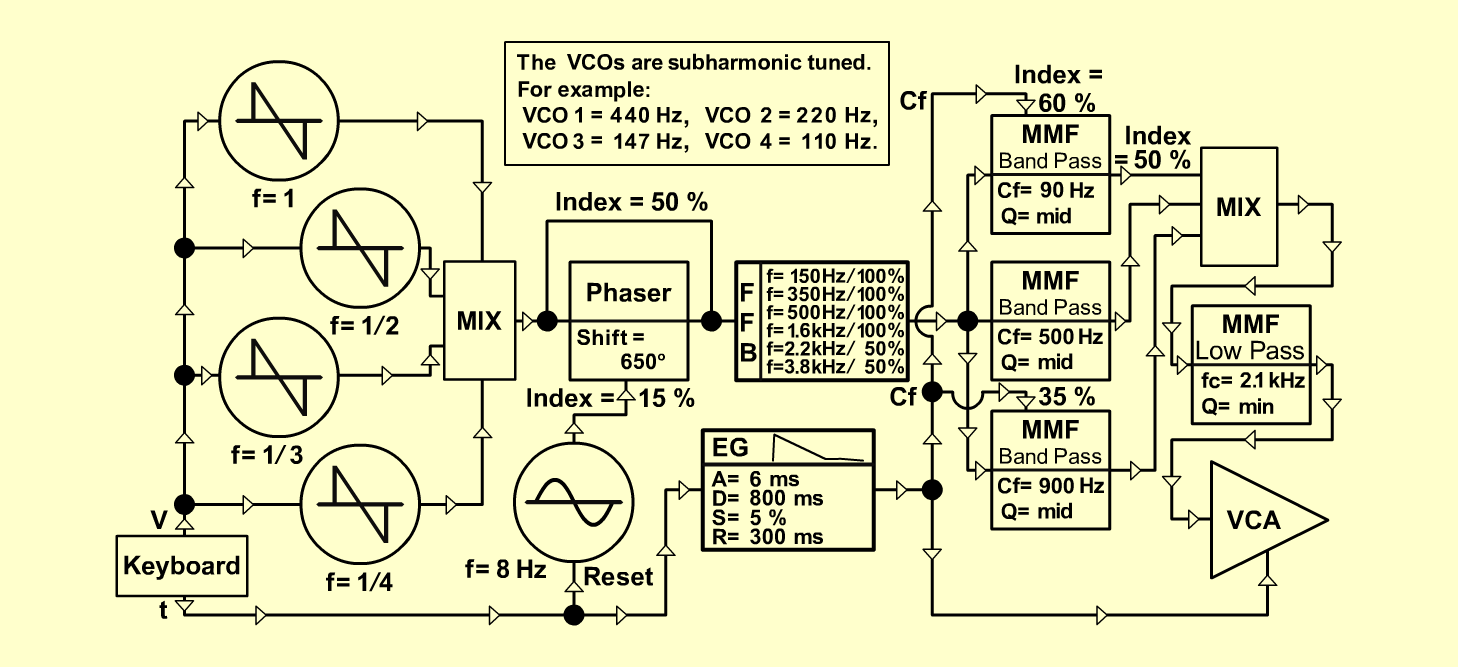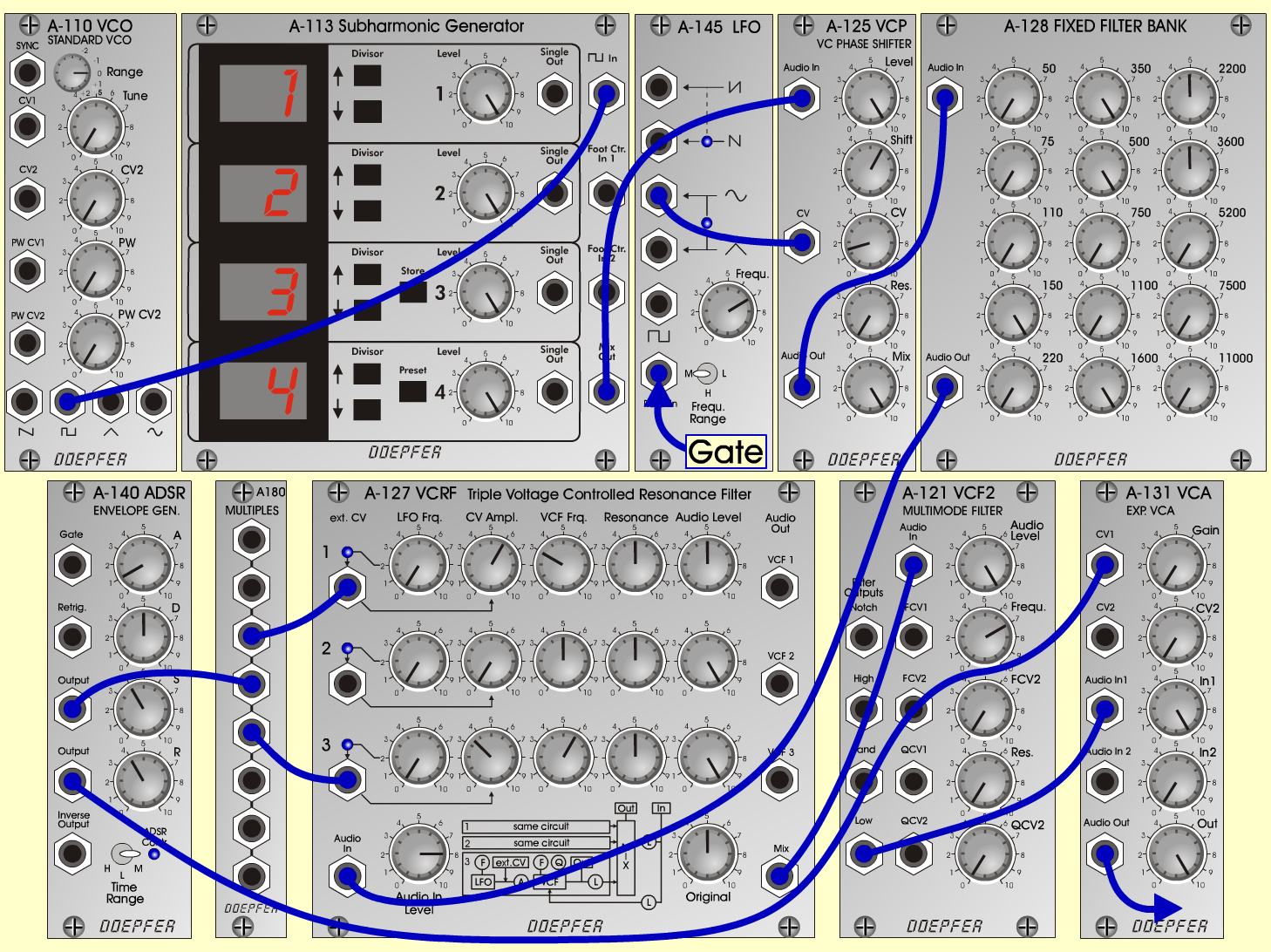ShanghaiS y n t hD o e p f e r A - 100 Connections: Settings: A-110 (Square)  <=>  A-113 (Square In) A-113 (Mix)  <=>  A-125 (Audio In) A-125 (Audio Out)  <=>  A-128 (Audio In) A-128 (Audio Out)  <=>  A-127 (Audio In) A-127 (Mix)  <=>  A-121 (Audio In) A-121 (Low)  <=>  A-131 (Audio In) A-145 (Sine)  <=>  A-125 (CV) A-140 (Output 1)  <=>  A-180 A-140 (Output 2)  <=>  A-131 (CV 1) A-180  <=>  A-127 (ext. CV 1) A-180  <=>  A-127 (ext. CV 3) Pitch, Trigger and Gate: KB KB - Gate  <=>  A-145 (Reset In) A-131 (Audio Out)  <=> Amplifier A-113 (Divisor 1 = 1, Divisor 2 = 2, Divisor 3 = 3, Divisor 4 = 4, Level 1 = 10, Level 2 = 10, Level 3 = 10, Level 4 = 10) A-125 (Level = 10, Shift = 6, CV = 1.5, Res = 0, Mix = 10) A-128 (50 = 0, 75 = 0, 110 = 0, 150 = 10, 220 = 0, 350 = 10, 700 = 10, 750 = 0, 1100 = 0, 1600 = 10, 2220 = 5, 3600 = 5, 5220 = 0, 7700 = 0, 11000 = 0) A-127 (Audio In = 8, Original = 5; Row 1: CV Ampl = 6, VCF Frq = 3, Res = 5, Audio Level = 5; Row 2: LFO Frq = 0, CV Ampl = 0, VCF Frq = 5, Res = 5, Audio Level = 10; Row 3: CV Ampl = 3.5, VCF Frq = 6, Res = 5, Audio Level = 10) A-121 (Audio Level = 10, Freq = 7, Res = 0) A-131 (Gain = 0, Audio In 1 = 10,  Audio Out = 10) A-145 (Frq = 7, Range = M) A-140 (A = 1, D = 5, S = 4, R = 4, Range = M) Notes: Instead of the A-113 (Subharmonic Generator) you can also take four VCOs with sawtooth-output (subharmonic tuned: f1 = 1, f2 = 1/2, f3 = 1/3, f4 = 1/4 - for example: VCO 1= 440 Hz, CVO 2 = 220 Hz, VCO 3 = 147 Hz, VCO 4 = 110 Hz). Josef MuellerSound samples Shanghai Melody Shanghai (261 Hz) Shanghai (523 Hz)